# Lecture Notes in Pattern Recognition: Classification vs Regression

These are the lecture notes for FAU’s YouTube Lecture “Pattern Recognition“. This is a full transcript of the lecture video & matching slides. We hope, you enjoy this as much as the videos. Of course, this transcript was created with deep learning techniques largely automatically and only minor manual modifications were performed. Try it yourself! If you spot mistakes, please let us know!

Welcome back to Pattern Recognition Q & A. Today I again received a couple of emails and I want to reply to some of your questions. So today’s topic will be classification and regression. We will see what are the differences between the two. We will look into a small regression problem and how to solve it with linear arguments.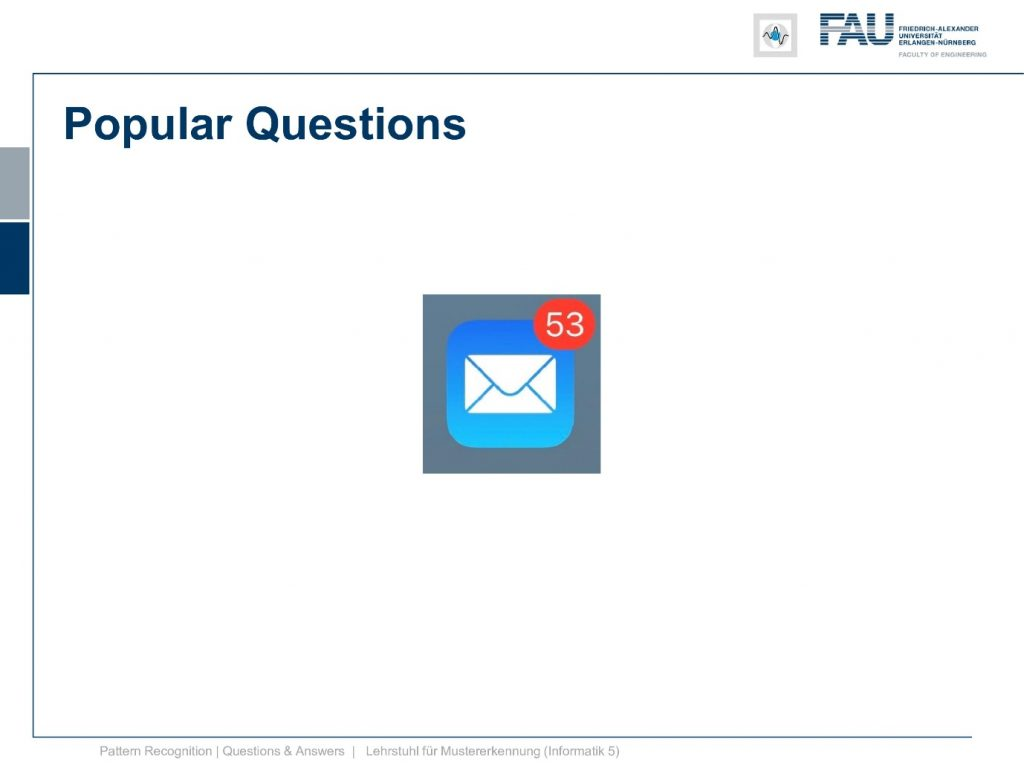Image under CC BY 4.0 from the Pattern Recognition Lecture.

So you send quite a few emails and you send many many questions and I had to select only a few of them. I also present some of the questions that I don’t want to actually answer in detail here. But those questions actually show up.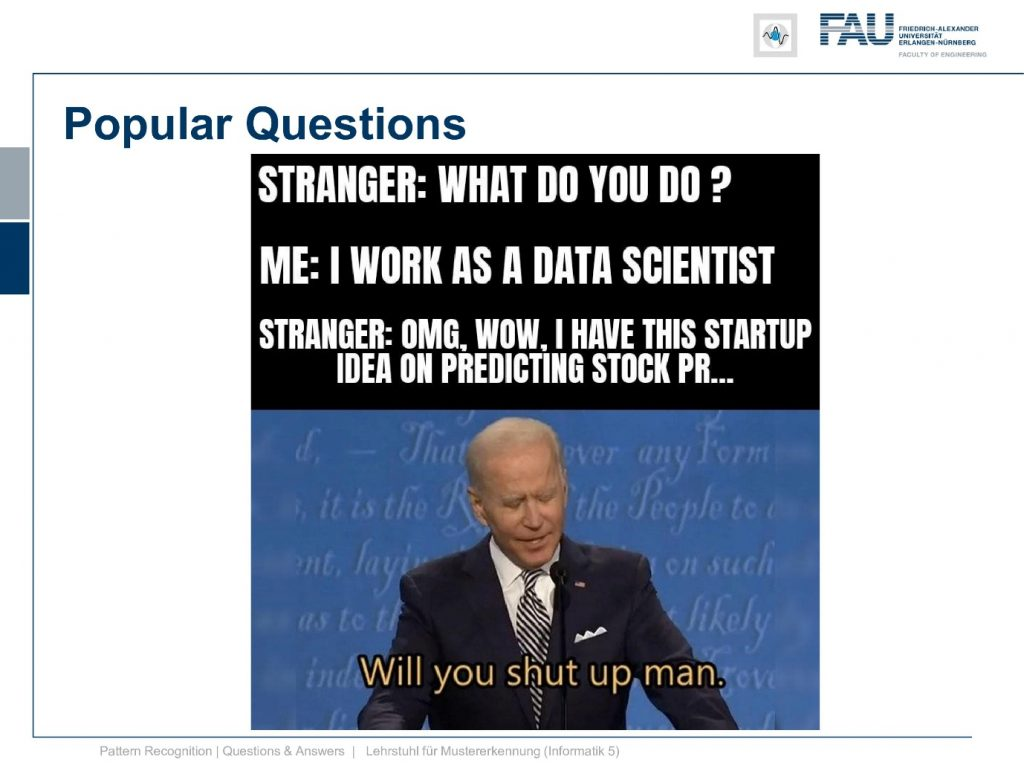Image under CC BY 4.0 from the Pattern Recognition Lecture.

One particular one is hey aren’t you a data scientist, a machine learning expert? I have this great idea about predicting stock price… Well, no I’m not gonna reply to such emails and I might even block you if you send stuff like this. So I won’t do any stock market price prediction and stuff like that. So you can save that email, I won’t answer that one.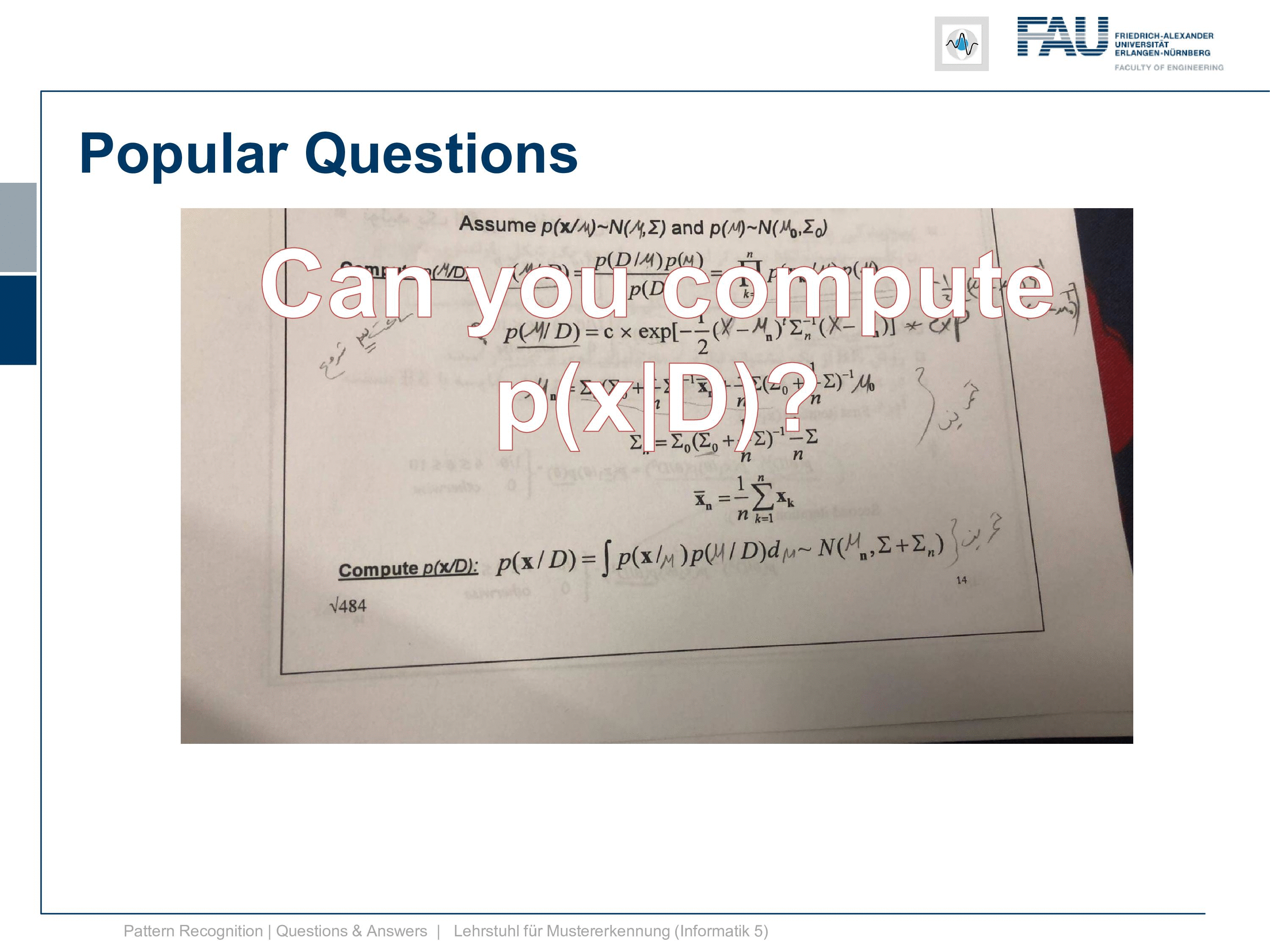Image under CC BY 4.0 from the Pattern Recognition Lecture.

Also questions like this one pop up. So can you compute p(x|d) and the answer is yes I can compute it? Then you ask something like can you send me the answer and the answer is no, I won’t do your homework and your home exercises. This is not what these videos are intended for in particular if they’re not even related to this class. I will answer of course questions that are related to the stuff that I talk about in these videos. I have now the class pattern recognition. I also have the class deep learning and I will answer about the stuff that we discuss in those videos. That’s perfectly fine. But I won’t solve your exercise problems. I won’t reply to those emails sorry.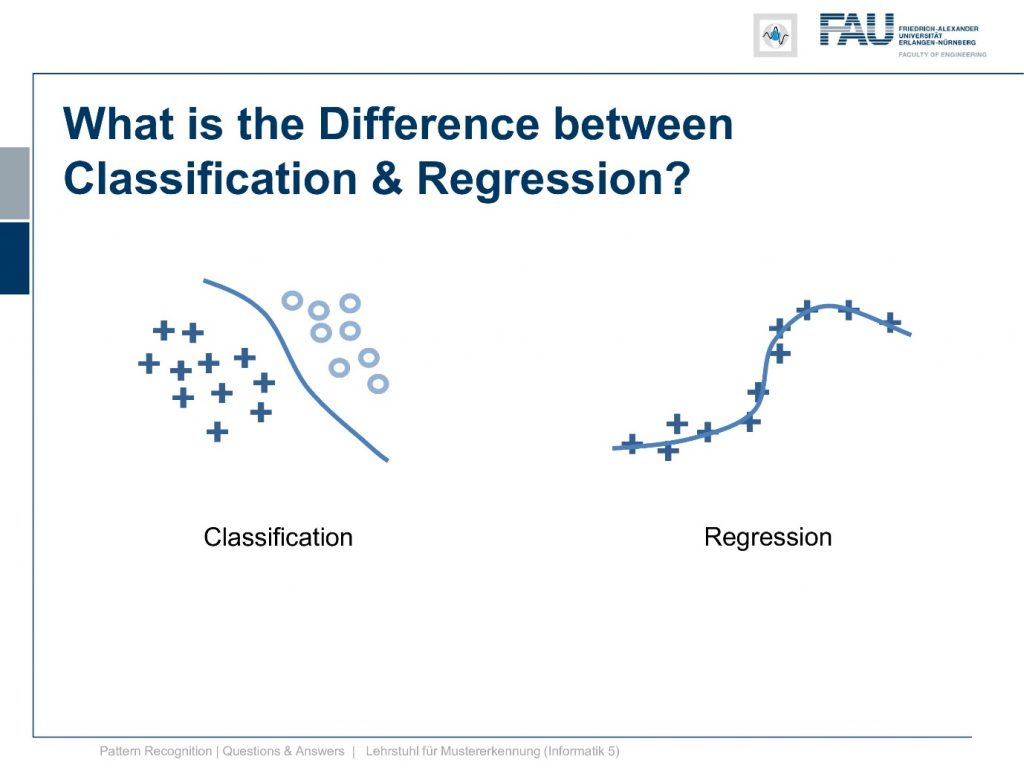Image under CC BY 4.0 from the Pattern Recognition Lecture.

Now let’s go into something where we really had a couple of questions and this actually concerns our class. One question that came up actually on a couple of occasions was what’s the difference actually between classification and regression. Now in classification, as we talked about in the first couple of videos we want to find the decision boundary and we actually assumed that the problem of regression is more or less known to you. So the problem of regression is actually trying to fit a certain mathematical construct. So let’s hear this is a kind of curve, maybe a polynomial, maybe it is also a spline or something like this and we try to fit it to the data such that it is able to explain the data and follow it. So this is a regression problem.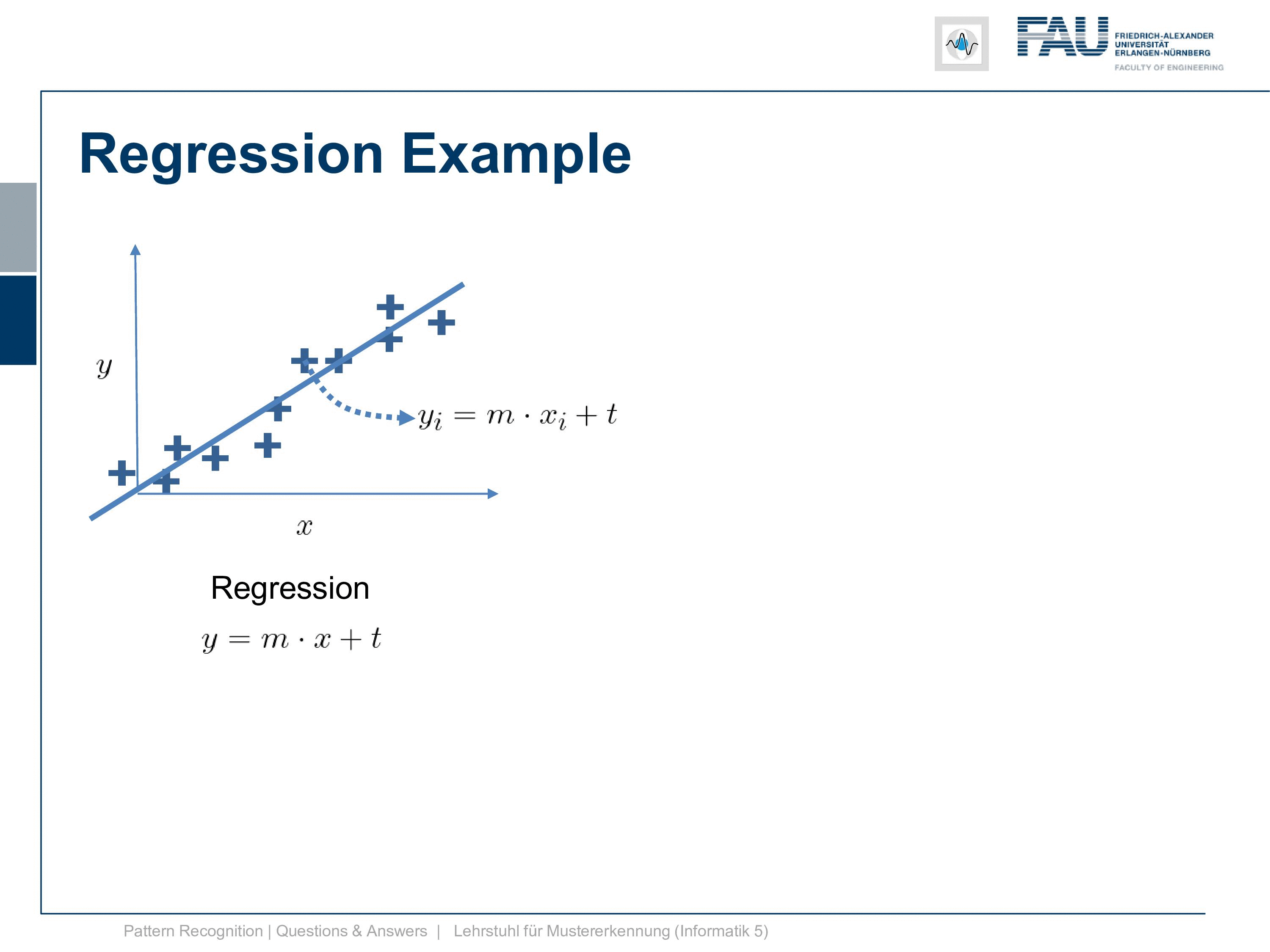Image under CC BY 4.0 from the Pattern Recognition Lecture.

What you encounter very frequently is linear regression. So in linear regression, we have a set of points and we want to fit a line through a set of points. Now you already see that I have an equation here. So this is the kind of model that we try to fit and we have the observed data x and y and we want to fit the unknown parameters m and t. Of course to do that I have to tell you what variable is what and this is of course here explained with the axis. So we have the y axis and the x-axis and now you can see that with every point I essentially have one observation of a pair of x and y values. Now what we try to find is the m and the t that match this observation best. You see that we have many of those observations. So for every point in the diagram, I actually get one equation. So I can rearrange this. Then we end up with a system of equations. You see I have the y1 that is then associated with the x1 and I have the yn where n is the number of points and this is associated with xn. So we have equations and now that we have many of those equations we can reformat this.

This thing is a system of linear equations. You can see here that the y’s can be written into a vector of y’s. Then we can separate the product and the sum on the right-hand side and we can express it with means of matrix multiplication. So here if you take the first line and you do the inner product with the vector mt and you assume in the current line. So let’s start with the first line. So this is y1 and x1 and now I expand this by essentially a column of ones in this matrix. You can see if I now compute the first line of this inner product I exactly end up with the first equation. if I do that for all of the other lines in the system of equations I exactly get the above equations that we already observed from the points. So we can rewrite this a little and you see that this can be expressed completely using matrix calculus.

So now we have a vector y, a matrix x, and some vector θ. What you see here is that we can observe all the y, we can observe all the x’s, of course also the ones because they just needed to be associated with the t. So everything is known in x and then y and all our unknowns are now in the parameter vector θ. What we now want to do is we want to solve the system of linear equations with respect to θ and we do that by computing absolute inverse. So the pseudo inverse is now multiplied to the vector y and this gives us an estimate of the parameter vector θ. So this would be a least-square solution and this least-square solution is typically found then as XTX this matrix inverted and then multiplied with XT. So this is the classical pseudo inverse. So you see this is a very important concept and it occurs all over the place in machine learning. I also found a nice meme for this.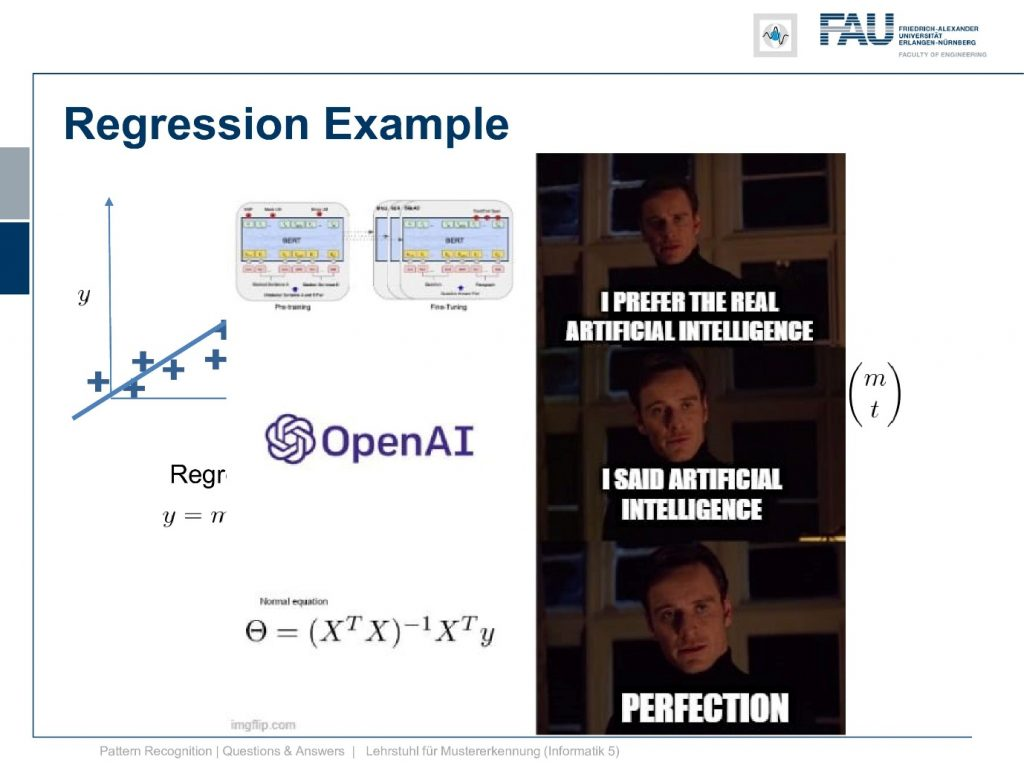Image under CC BY 4.0 from the Pattern Recognition Lecture.

So there’s all the fancy kind of network architectures and so on and of course we want to have real artificial intelligence. Then there’s OpenAI and they also offer a lot of tools. Here we of course want to save artificial intelligence and then you see most of the time you then just apply a kind of pseudo-inverse and linear regression. You can solve these things quite easily. Well, that’s not actually the entire thing but with linear regression, you already get pretty far. If you know how to set up the matrixes correctly then you can even fit polynomials and so on. So linear regression is a very very nice concept and it’s very useful in practical use. It’s very frequently employed.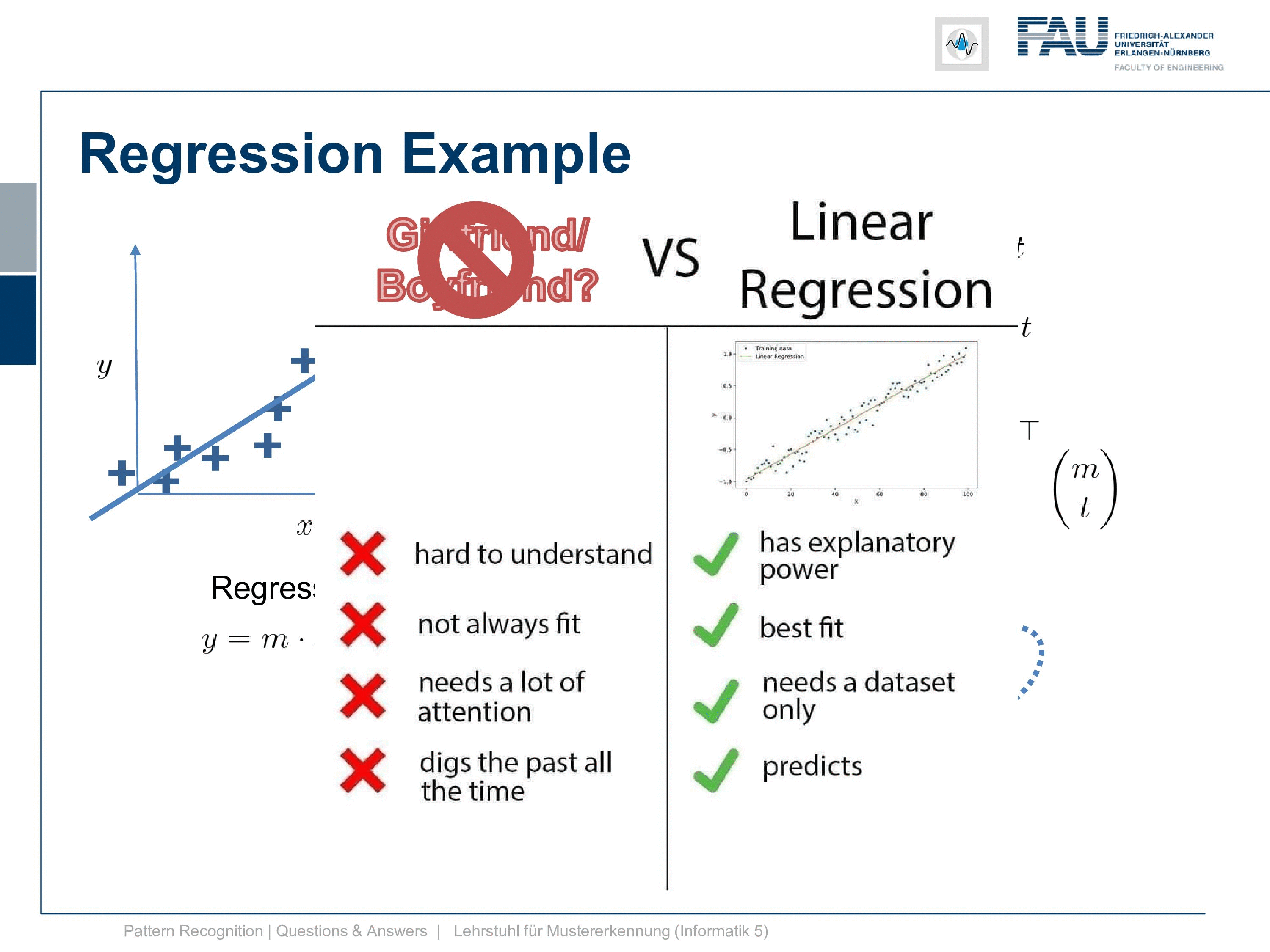Image under CC BY 4.0 from the Pattern Recognition Lecture.

So I have a couple of points that are very useful about linear regression. So let’s compare it and you see it has explanatory power it produces the best fit it only needs a data set and then it predicts. So this is a very cool technique. So what I’m comparing to here is it your boyfriend girlfriend. Well, no, it’s actually this guy here.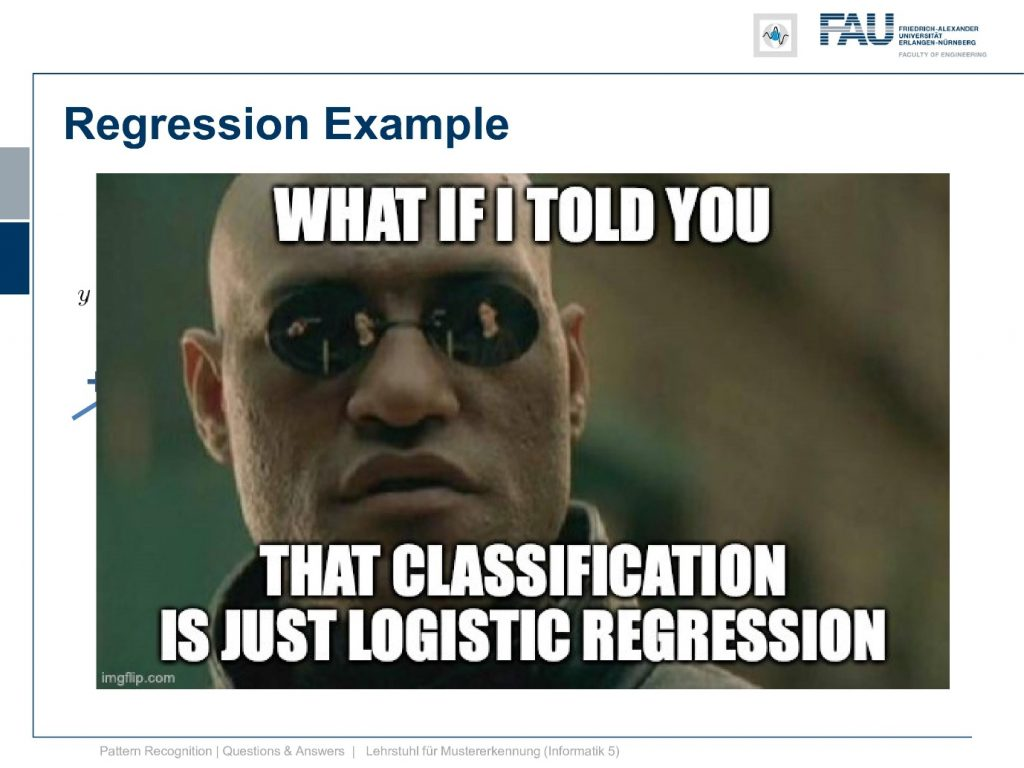Image under CC BY 4.0 from the Pattern Recognition Lecture.

So what we’ve also seen in the lecture and if you look at the respective episode you can see then that even the concept of classification can be expressed in terms of regression. Because where we are directly fitting the decision boundary by using the logistic function. So classification is just logistic regression.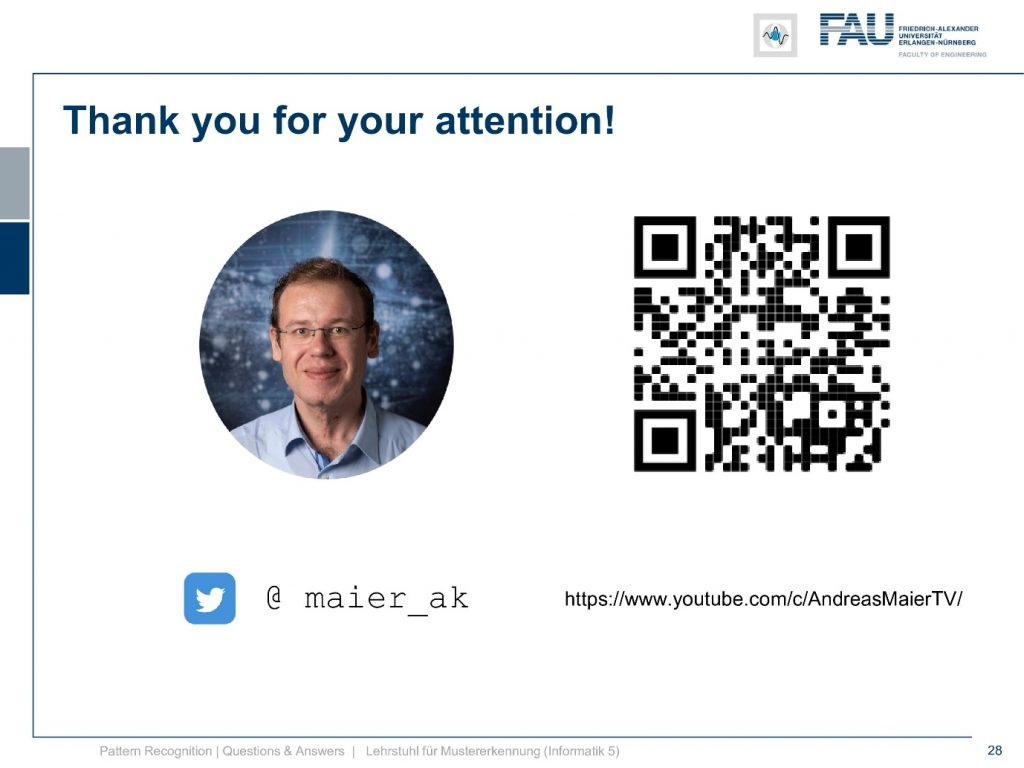Image under CC BY 4.0 from the Pattern Recognition Lecture.

So thank you very much for listening and of course, I’d be very happy to answer more of your questions. So you can send them, you can leave them as comments, you can also interact with me on social media. So I also put the links in the description of this video. I’m very much looking forward to your questions and I take time to answer them if they are related to my class. Otherwise, I might just ignore them. So thank you very much for watching and looking forward to seeing you in one of the next videos. Bye-bye!!

If you liked this post, you can find more essays here, more educational material on Machine Learning here, or have a look at our Deep Learning Lecture. I would also appreciate a follow on YouTubeTwitterFacebook, or LinkedIn in case you want to be informed about more essays, videos, and research in the future. This article is released under the Creative Commons 4.0 Attribution License and can be reprinted and modified if referenced. If you are interested in generating transcripts from video lectures try AutoBlog